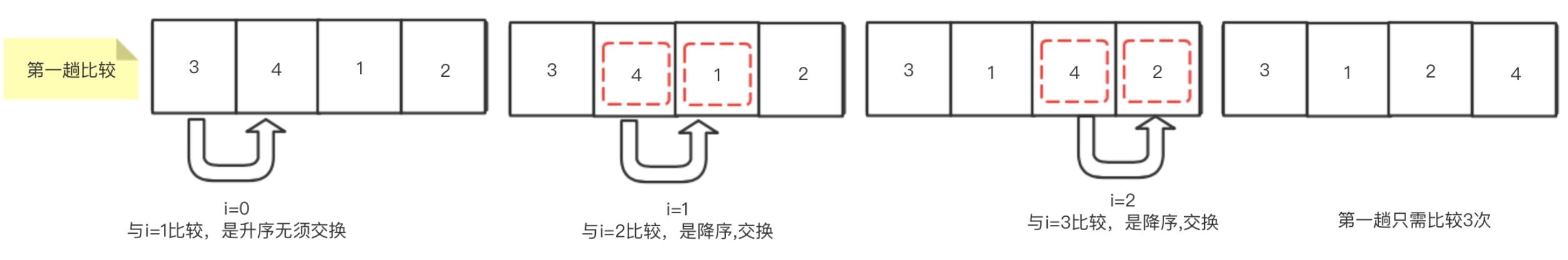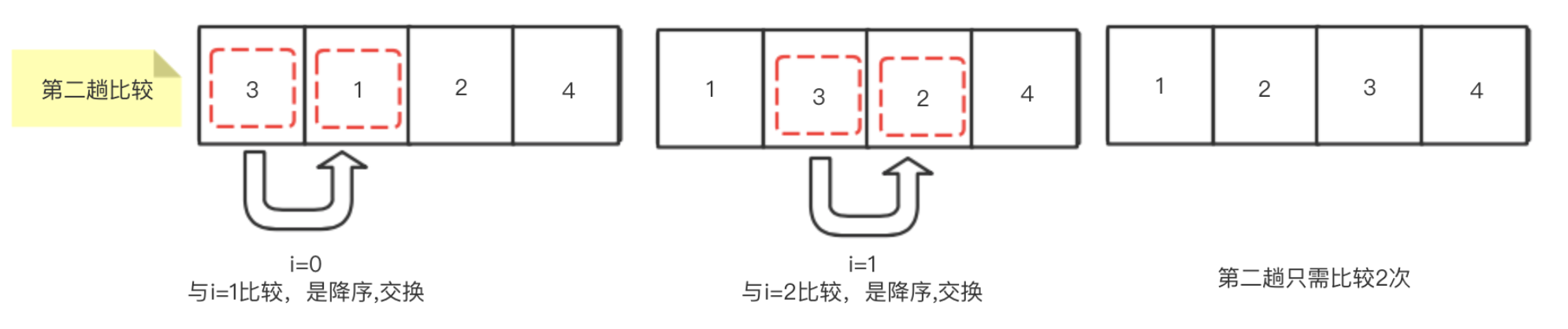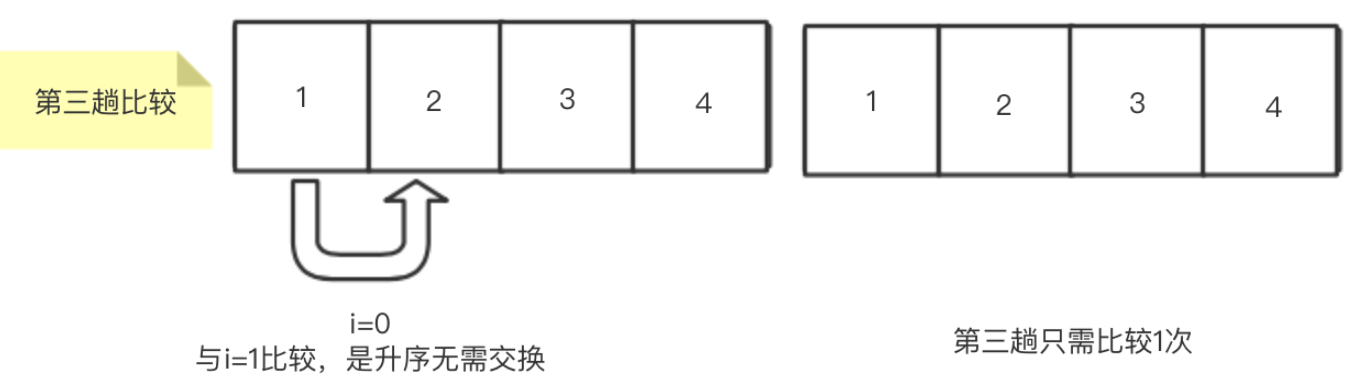Categories

# Classical sorting algorithms – Bubble Sort

Bubble sort of principle

Bubble sort principle starting from the first number, so that the two numbers are sequentially comparing adjacent, according to descending or ascending order of exchange (in ascending order if it is put in front of the small discharge, if descending put in front of a large discharge).

Comparing the first pass, the maximum number put in the last position (assuming ascending order), and then comparing the second pass, sequentially adjacent to the digital comparator, a second comparator trip time after a large number in the penultimate position.

After comparing trip n-1 (n representative of the number of digits to be sorted) (only the last without a comparison), is the number ordered.

Graphic shows the process of bubbling

Number is assumed to be arranged in 3142, when the first trip sort, as shown below (where the subscript i denotes the array)Sort first trip after the implementation, the array largest number 4 has been found and placed in the last position after the array, so no need to talk to the last follow-up compare comparison. Let’s look at the second pass the sorting process, as shown belowSort by executing the second trip, the second largest digital array 3 has been found and placed in the penultimate position of the array. At this time, since we selected arrangement of data coincidence, also the third largest number 2 position in the bottom third, but according to our comparison logic, does not know at this time the third largest numbers have found, so it will be the third Compare trip. As shown belowAccording to our logic, when the comparison is performed after the third trip, the third largest number 2 has been found and placed third in the inverse position. The last remaining position number 1 do not need to compare its own position is that it should be located.

We can see that when there are four figures, only need 3 times the disorder can sort numbers into order, and each trip will be relatively less than once a relatively time (because the trip has identified a more compare large digits)

Further, in our example, third Comparing times already in the correct sequence number, so no further subsequent comparison (of course, we only compare just three times, if there are five or more digits, may be omitted subsequent comparison)

Let’s look at the specific code implementation

``` 1 public static void bubbleSort(int array[]){
2         int temp = 0;
3         for (int i = 0; i < array.length - 1; i++) {
4             //There are n data, only n-1 times to sort
5             boolean flag = true;
6             for(int j=0;j){
7                 if(array[j] > array[j+1]){
8                     flag = false;
9                     temp = array[j];
10                     array[j] = array[j+1];
11                     array[j+1] = temp;
12                 }
13             }
14             if(flag){
15                 break;
16             }
17         }
18     }```

Code analysis

1) a first layer for loop several times for determining a comparison, we have analyzed the front, just for comparison may be n-1 times

2) The second layer for loop is used to start with the first number and compare with the adjacent number in turn. Compare one digit less for each trip

3) performing the comparison cycle of the second layer, In Flag set a flag, used to indicate whether or not the digital switching, if not once switched, description has been ordered number this time, without the need for a subsequent comparison of the times

time complexity

Bubble sort two nested sorting cycle, so its time complexity is T (n) = O (n ^ 2).

Test Execution Time Code

Here we generate an array of 100,000 random numbers, using the bubble sort method to sort, to see the execution time. In my follow-up article, we will continue to share several other sorting algorithms, and will also use 100 000 random numbers calculated in several other sort of time sorting algorithms.

``` 1 public static void main(String []args){
2         int array[] = new int;
3         bubbleSort(array);
4         for(int i=0; i<100000; i++){
5             array[i] = (int)(Math.random()*1000000);
6         }
7         long begin = System.currentTimeMillis();
8         bubbleSort(array);
9         System.out.println("总耗时="+(System.currentTimeMillis()-begin));
10     }```

Execution result (in milliseconds)It can be seen for 100,000 to sort the data using a bubble sort algorithm on our machine takes about 17 seconds time. Next I will share selected sorting algorithm, choose whether to perform time sorting algorithm will reduce it? Together we look forward to it!

to sum up

Bubble sort to keep in mind three points, one compares the number of times n-1 (the last number without having to compare), and second, it will determine the position of a higher number after every trip to the comparison, the number of comparisons will be the follow-up trip one less. Another point is to optimize the point, when the comparison has been generated in the correct order of the digital process, no longer need to be compared to subsequent times.